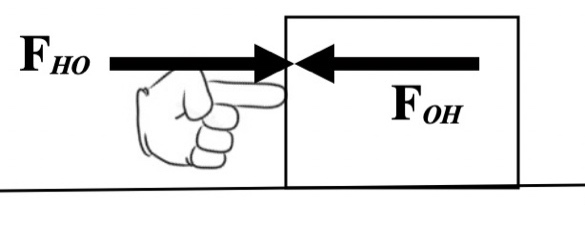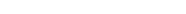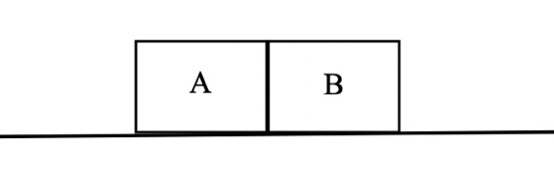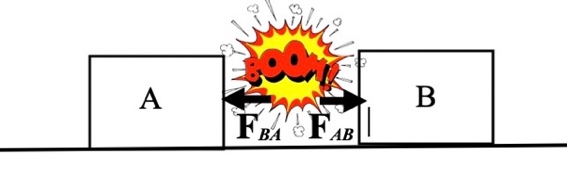Interactive Lecture Demonstrations

# Prediction Sheet--Newton's  3rd Law

Directions:  Click here to download the Prediction Sheet on which you will record your predictions.  Write your name at the top to record your presence and participation in these demonstrations.  For each demonstration, write your prediction on this sheet before making any observations. You may be asked to send this sheet to your instructor.

 Demonstrations 1, 2, 3: Someone pushes an object on a smooth surface. The block experiences a constant frictional force opposite to its motion.  Compare the following two forces in magnitude and direction, FHO (the force of the Hand on the Object) and FOH (the force of the Object on the Hand) during each of the three demonstrations described below. Demonstration 1:  The object is being pushed at a constant velocity. Predict how the force FHO compares FOH. How does the magnitude of FHO compare to the force of friction?  What is the net force on the object? Demonstration 2: The object is pushed so that it speeds up. Predict how the force FHO compares to FOH. How does the magnitude of FHO compare to the force of friction?  What is the net force on the object? Demonstration 3: The block is pushed so that it slows down. Predict how the force FHO compares to FOH. How does the magnitude of FHO compare to the force of friction?  What is the net force on the object? Only after you have made all of your predictions, click here to view a video of one object being pushed by another. Force sensors measure the two forces. In each case, observe the magnitudes of the two forces, and compare to your predictions. Explain any differences.Demonstration 4:  Two identical carts (called Car A and Car B) are given pushes so that they move toward each other at the same speed.  Predict how FAB (the force of Car A on Car B) compares to FBA (the force of Car B on Car A) during the collision.  How do the directions of the forces compare? Only after you have made your predictions, click here to view a video of the situation. Observe the two force and compare to. your predictions. Explain and differences. Demonstration 5:  A cart is given a push so that it moves toward an identical cart that is at rest (called Car A and Car B). Predict how FAB (the force of Car A on Car B) compares to FBA (the force of Car B on Car A) during the collision.  How do the directions of the forces compare? Only after you have made your predictions, click here to view a video of the situation. Observe the two force and compare to. your predictions. Explain and differences. Demonstration 6:  A massive (heavy) cart (cart A--like a truck) is given a push so that it moves toward a light cart (cart B--like a small car) that is at rest. Predict how FAB (the force of the heavy truck on the small car) compares to FBA (the force of the small car on the heavy truck) during the collision.  How do the directions of the forces compare? Only after you have made your predictions, click here to view a video of the situation. Observe the two force and compare to. your predictions. Explain and differences. Demonstration 7: Two identical objects A and B (same mass) are sitting against each other on a level surface. There is an explosive charge between them. The charge is set off, and the two objects move apart. During the explosion, predict how the force FAB exerted by object A on object B during the explosion compares to the force FBA exerted by object B on object A. Only after you have made your predictions, click here to view a video of the situation. Observe the two force and compare to. your predictions. Explain and differences.Demonstration 8: In Demonstration 7, suppose that object A is much more massive (heavier) than object B. When the charge is set off, the two objects again move apart. During the explosion, predict the force FAB exerted by object A on object B to the force FBA exerted by object B on object A. Only after you have made your predictions, click here to view a video of the situation. Observe the two force and compare to. your predictions. Explain and differences.